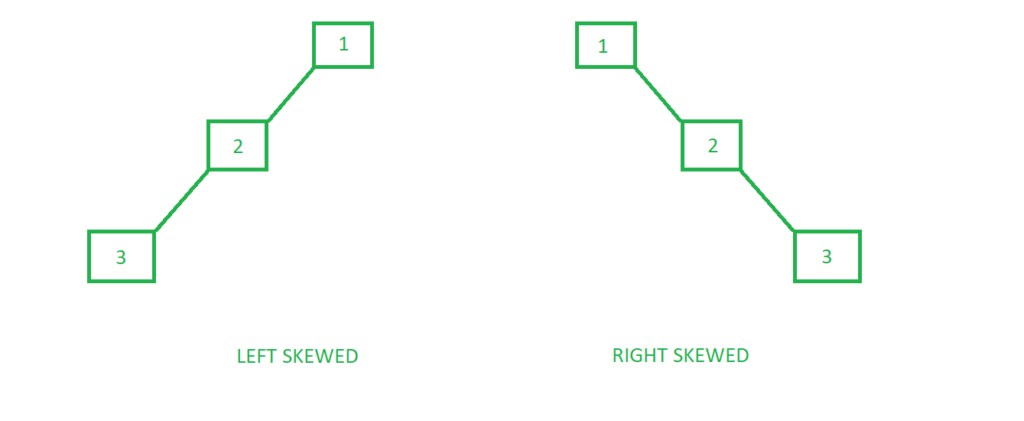# Skewed Binary Tree

A skewed binary tree is a type of binary tree in which all the nodes have only either one child or no child.

Types of Skewed Binary trees
There are 2 special types of skewed tree:1. Left Skewed Binary Tree:
These are those skewed binary trees in which all the nodes are having a left child or no child at all. It is a left side dominated tree. All the right children remain as null.

Below is an example of a left-skewed tree:

## C++

 `#include ` `using` `namespace` `std; ` ` `  `// A Tree node ` `struct` `Node { ` `    ``int` `key; ` `    ``struct` `Node *left, *right; ` `}; ` ` `  `// Utility function to create a new node ` `Node* newNode(``int` `key) ` `{ ` `    ``Node* temp = ``new` `Node; ` `    ``temp->key = key; ` `    ``temp->left = temp->right = NULL; ` ` `  `    ``return` `(temp); ` `} ` ` `  `// Driver code ` `int` `main() ` `{ ` `    ``/* ` `            ``1 ` `           ``/ ` `          ``2 ` `         ``/ ` `        ``3 ` `    ``*/` `    ``Node* root = newNode(1); ` `    ``root->left = newNode(2); ` `    ``root->left->left = newNode(3); ` ` `  `    ``return` `0; ` `} `

## Java

 `// Java implementation of above approach ` `import` `java.util.*; ` ` `  `class` `GFG ` `{ ` `     `  `// A Tree node ` `static` `class` `Node  ` `{ ` `    ``int` `key; ` `     ``Node left, right; ` `}; ` `   `  `// Utility function to create a new node ` `static` `Node newNode(``int` `key) ` `{ ` `    ``Node temp = ``new` `Node(); ` `    ``temp.key = key; ` `    ``temp.left = temp.right = ``null``; ` `   `  `    ``return` `(temp); ` `} ` `   `  `// Driver code ` `public` `static` `void` `main(String args[]) ` `{ ` `    ``/* ` `            ``1 ` `           ``/ ` `          ``2 ` `         ``/ ` `        ``3 ` `    ``*/` `    ``Node root = newNode(``1``); ` `    ``root.left = newNode(``2``); ` `    ``root.left.left = newNode(``3``); ` `} ` `} ` ` `  `// This code is contributed by Arnab Kundu `

## C#

 `// C# implementation of above approach  ` `using` `System; ` `  `  `class` `GFG  ` `{  ` `          `  `    ``// A Tree node  ` `     ``public` `class` `Node  ` `    ``{  ` `         ``public` `int` `key;  ` `         ``public` `Node left, right;  ` `    ``};  ` `          `  `    ``// Utility function to create a new node  ` `     ``static` `Node newNode(``int` `key)  ` `    ``{  ` `        ``Node temp = ``new` `Node();  ` `        ``temp.key = key;  ` `        ``temp.left = temp.right = ``null``;  ` `          `  `        ``return` `(temp);  ` `    ``}  ` `          `  `    ``// Driver code  ` `    ``public` `static` `void` `Main()  ` `    ``{  ` `        ``/*  ` `                ``1  ` `            ``/  ` `            ``2  ` `            ``/  ` `            ``3  ` `        ``*/` `        ``Node root = newNode(1);  ` `        ``root.left = newNode(2);  ` `        ``root.left.left = newNode(3);  ` `    ``}  ` `} ` ` `  `// This code is contributed by AnkitRai01 `

2. Right Skewed Binary Tree:
These are those skewed binary trees in which all the nodes are having a right child or no child at all. It is a right side dominated tree. All the left children remain as null.

Below is an example of a right-skewed tree:

## C++

 `#include ` `using` `namespace` `std; ` ` `  `// A Tree node ` `struct` `Node { ` `    ``int` `key; ` `    ``struct` `Node *left, *right; ` `}; ` ` `  `// Utility function to create a new node ` `Node* newNode(``int` `key) ` `{ ` `    ``Node* temp = ``new` `Node; ` `    ``temp->key = key; ` `    ``temp->left = temp->right = NULL; ` ` `  `    ``return` `(temp); ` `} ` ` `  `// Driver code ` `int` `main() ` `{ ` `    ``/* ` `        ``1 ` `         ``\ ` `          ``2 ` `           ``\ ` `            ``3 ` `    ``*/` `    ``Node* root = newNode(1); ` `    ``root->right = newNode(2); ` `    ``root->right->right = newNode(3); ` ` `  `    ``return` `0; ` `} `

## Java

 `// Java implementation of above approach ` `import` `java.util.*; ` `class` `GFG ` `{ ` `     `  `// A Tree node ` `static` `class` `Node ` `{ ` `    ``int` `key; ` `    ``Node left, right; ` `}; ` `   `  `// Utility function to create a new node ` `static` `Node newNode(``int` `key) ` `{ ` `    ``Node temp = ``new` `Node(); ` `    ``temp.key = key; ` `    ``temp.left = temp.right = ``null``; ` `   `  `    ``return` `(temp); ` `} ` `   `  `// Driver code ` `public` `static` `void` `main(String args[]) ` `{ ` `    ``/* ` `       ``1 ` `        ``\ ` `         ``2 ` `          ``\ ` `           ``3 ` `    ``*/` `    ``Node root = newNode(``1``); ` `    ``root.right = newNode(``2``); ` `    ``root.right.right = newNode(``3``); ` `} ` `} ` ` `  `// This code is contributed by Arnab Kundu `

## C#

 `// C# implementation of above approach ` `using` `System; ` `  `  `class` `GFG ` `{ ` `       `  `// A Tree node ` `public` `class` `Node ` `{ ` `    ``public` `int` `key; ` `    ``public` `Node left, right; ` `}; ` `     `  `// Utility function to create a new node ` `static` `Node newNode(``int` `key) ` `{ ` `    ``Node temp = ``new` `Node(); ` `    ``temp.key = key; ` `    ``temp.left = temp.right = ``null``; ` `     `  `    ``return` `(temp); ` `} ` `     `  `// Driver code ` `public` `static` `void` `Main(String []args) ` `{ ` `    ``/* ` `       ``1 ` `        ``\ ` `         ``2 ` `          ``\ ` `           ``3 ` `    ``*/` `    ``Node root = newNode(1); ` `    ``root.right = newNode(2); ` `    ``root.right.right = newNode(3); ` `} ` `} ` `  `  `// This code is contributed by PrinciRaj1992 `

My Personal Notes arrow_drop_upCheck out this Author's contributed articles.

If you like GeeksforGeeks and would like to contribute, you can also write an article using contribute.geeksforgeeks.org or mail your article to contribute@geeksforgeeks.org. See your article appearing on the GeeksforGeeks main page and help other Geeks.

Please Improve this article if you find anything incorrect by clicking on the "Improve Article" button below.